How can extremism prevail? A study based on the relative agreement interaction model

Journal of Artificial Societies and Social Simulation vol. 5, no. 4
<https://www.jasss.org/5/4/1.html>

To cite articles published in the Journal of Artificial Societies and Social Simulation, please reference the above information and include paragraph numbers if necessary

Received: 17-Jul-2002      Accepted: 20-Sep-2002      Published: 31-Oct-2002

###Abstract

Abstract: We model opinion dynamics in populations of agents with continuous opinion and uncertainty. The opinions and uncertainties are modified by random pair interactions. We propose a new model of interactions, called relative agreement model, which is a variant of the previously discussed bounded confidence. In this model, uncertainty as well as opinion can be modified by interactions. We introduce extremist agents by attributing a much lower uncertainty (and thus higher persuasion) to a small proportion of agents at the extremes of the opinion distribution. We study the evolution of the opinion distribution submitted to the relative agreement model. Depending upon the choice of parameters, the extremists can have a very local influence or attract the whole population. We propose a qualitative analysis of the convergence process based on a local field notion. The genericity of the observed results is tested on several variants of the bounded confidence model.

Keywords:
Extremists; Individual-based Simulation; Opinions Dynamics

###Introduction

1.1
How can opinions, which are initially considered as extreme and marginal, manage to become the norm in large parts of a population? Several examples in the world history show that large communities can more or less suddenly switch globally to one extreme opinion, because of the influence of an initially small minority. Germany in the thirties is a particularly dramatic example of such a process. In the last decades, an initial minority of radical Islamists managed to convince large populations in Middle East countries. But one can think of less dramatic processes, like fashion for instance, where the behaviour of minority groups, once considered as extremist, becomes the norm in a large part of the population (it is the case of some gay way of dressing for instance). On the other hand, one can also find many examples where a very strong bipolarisation of the population takes place, for instance the cold war period in Europe. In these cases, the whole population becomes also extremist (choosing one side or the other). Of course, the existence of limited groups of extremists who do not have a global influence on the whole population is also a quite common situation. The influence of "green" farmers in the farming population, which originally motivated this work, can be an example of such a situation.

1.2
Several attempts to build related models were proposed, but they generally consider binary opinions and mostly concern the resistance of a minority to maintain itself or its power (see for instance Latané and Nowak 1997; Galam 1997; Galam and Wonczak 2000; Kacpersky and Holyst 2000). In these models, because the opinions are binary, it is impossible to distinguish between moderate and extreme opinions (we have only minority or majority opinions). In terms of building models, the more or less progressive drift from moderate to extreme opinion is of course more challenging and this is the approach we take here. This is the reason why we consider continuous opinion dynamics. Moreover, we are interested in a very rough interpretation of the general context (political, economic or social conditions prevailing during the rise of extremism). This interpretation is given in our model by the uncertainty, which is related to each opinion. The context of crisis, favourable to the rise of extremism, corresponds to very high uncertainties.

1.3
We propose here a very stylised opinion dynamics model in which, according to parameter values, an evolution to extremism can occur. For other parameter values the majority resists the influence of extremists. We are particularly interested in the conditions that lead to one or the other situation.

1.4
The model is an extension of the "Bounded Confidence" (BC) model (Krause 2000; Deffuant et al. 2000; Dittmer 2001; Hegselmann and Krause 2002). In the BC model, the agents have continuous opinions and the interactions are non-linear: the agents actually influence each other only if the distance between their opinions is below a threshold. The threshold can be interpreted as an uncertainty, or a bounded confidence, around the opinion. It is assumed that people do not take into account opinions out of their range of uncertainty.

1.5
Because of the threshold condition, the BC model can be seen as a non-linear version of previous continuous opinion models (Chatterjee and Seneta 1977; Cohen et al. 1986; Friedkin and Johnsen 1990; Friedkin and Johnsen 1999).

1.6
In this paper, we propose an extension of the BC model, which we call the relative agreement (RA) model. As in our previous version of the BC model, opinions are adjusted during pair interactions, but several differences are noticeable.

1.7
The main assumption of the model is that the influence of an agent on a partner is linear with the relative overlap (agreement) between their opinion segments. This leads to the following noticeable difference with the BC model:
• The influence varies as the inverse of the influencing agent uncertainty, which makes the extremists (less uncertain according to our hypotheses) more influential,
• There is a continuous variation of the influence according to the distance between the opinions (which is not the case in the BC model)

1.8
Moreover, during interactions, the agents influence each other's uncertainties as well as each other's opinions.

1.9
We study the RA model behaviour on initial uniform distributions of opinions in which we introduce a set of extremists: a small proportion of agents with the most extreme opinions have a much lower uncertainty than the majority.

1.10
The study is limited to the case where all the agents can a priori interact with each other (full connection rather than a network of interaction).

1.11
In some parameter zones, the influence of the extremists is very limited. However, in other parameter zones, the whole population becomes extremist, sometimes with a balanced number in both extremes, sometimes one extreme being largely dominant. This last situation, in which one extreme prevails, was unexpected. However, it can be also found with the BC model. The bipolarisation to the extremes is more specific to the RA model (it can almost never be obtained with the BC model).

1.12
In section 2, we present the RA model and the type of convergence obtained when the whole population has an identical uncertainty. In section 3, we describe the model with extremists and the different dynamical regimes. Section 4 presents the simulation results and the exploration of parameter space. Section 5 proposes a more insightful analysis of the convergence process on representative examples. In section 6 we compare simulation results for both models, RA and BC, in the presence of extremists.

###The Relative Agreement model

#### Model definition

2.1
We consider a population of N agents. Each agent i is characterised by two variables, its opinion xi and its uncertainty ui, both being real numbers. We call segment of the opinion axis the opinion segment of agent i. In the following, we suppose that opinions are drawn from a uniform distribution between -1 and +1.

2.2
The relative agreement model can be seen as an extension of the BC model. We suppose that random pair interactions take place among the agents, during which they may influence each other's opinion segment. The main difference between the models is in the way the RA model takes into account the uncertainty in opinion dynamics: the change in opinion xj of agent j under the influence of agent i is proportional to the overlap between both segments (the agreement), divided by the uncertainty of the influencing segment (which explains why we call it "relative"). Note that the actual influence is non-symmetrical when the agents have different uncertainties, because of the division by the uncertainty of the influencing agent (see figure 1). After agents i and j opinion and uncertainty updating, a new pair is randomly chosen and the same process is iterated until attractors of the dynamics with invariant opinions and uncertainties are reached.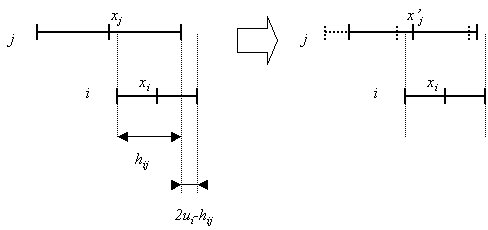Figure 1. agent i (opinion xI and uncertainty uI) influences agent j (opinion xj and uncertainty uj). hij is the overlap between i and j and 2ui-hij is the part of i non-overlapping with j. On the right, the dotted lines represent the position of the segment before the interaction, and plain lines after.

2.3
More precisely, let us consider opinion segments si=[xi-Ui, Xi+Ui] and sj=[xj-Uj, Xj+Uj]. We define the agreement of agent i with j (it is not symmetric) as the overlap of sI and sj, minus the non-overlapping part (see figure 1).
The overlap hij is given by: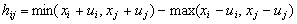(Eq. 1)
The non-overlapping width is: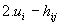(Eq. 2)
The agreement is the overlap minus the non-overlap: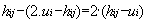(Eq. 3)
The relative agreement is the agreement divided by the length of segment si: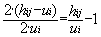(Eq. 4)

2.4
If hij > ui, then the modification of xj and uj by the interaction with i is very similar to the ones of the bounded confidence dynamics, except that the modifications are multiplied by the relative agreement: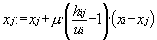(Eq. 5)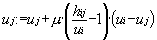(Eq. 6)
where µ is a constant parameter which amplitude controls the speed of the dynamics. If hijui, there is no influence of i on j.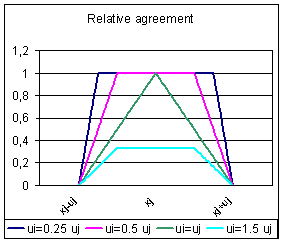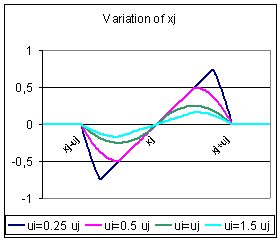Figure 2. On the left, plot of the relative agreement of i with j as a function of xi, for different values of ui. On the right, plot of the variation of xj as a function of xi, for different values of ui. When ui tends to 0, the relative agreement tends to be a window. When ui > 2uj the relative agreement is 0 and i has no influence on j. The variation of xj is null when xi and xj are equal. When the distance between xi and xj increases, the variation of xj increases until a maximum and then decreases.

2.5
The main features of the relative agreement model are:
• During interactions, agents not only influence each other's opinions but also each other's uncertainties.
• The influence is not symmetric when the agents have different uncertainties (see figure 2), "confident" agents (low uncertainty) are more influential. This corresponds to the common experience in which confident people tend to convince more easily uncertain people than the opposite (when their opinions are not too far apart).
• The influence (the modifications of xj and uj) varies continuously when xj, uj, xi and ui vary continuously (see figure 2). On the contrary, in the BC model the modification falls down to zero suddenly when xj crosses the threshold distance. This sudden change of influence after the peak is difficult to justify from a psychological point of view.

#### Case of identical uncertainties in the whole population

2.6
The general behaviour is similar to the BC model: the local maxima of the density of opinions tend to be amplified by absorbing the opinions within the distance u. The population converges into a set of clusters of the same opinion, more or less equally distributed (depending on the initial distribution of local maxima). An example of evolution of the population in the case of initial identical uncertainties is represented on figure 3.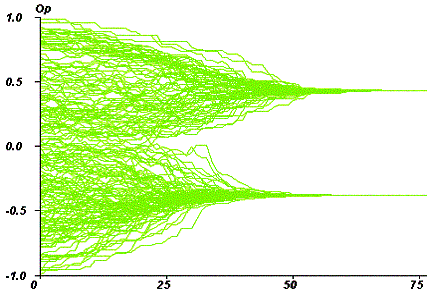Figure 3. Time chart of opinions for an initial uniform uncertainty of 0.4. The time scale is the average number of iterations per agent. N, the agents umber is 200.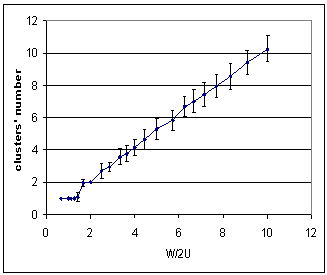Figure 4. Plot of the average cluster number as a function of w/2u (w is the width of the initial distribution and u is the uncertainty of the agents). Each dot represents the final number of clusters averaged over 50 simulations (an isolated agent is not considered as a peak). The number of clusters is close to w/2u (r2=0.99), whereas it is close to the integer part of w/2u in the BC model.

2.7
In the case of a constant initial uncertainty u in the whole population, and for an initial uniform distribution of opinions of width w, the average number of clusters is close to w/2u (see figure 4). There is a slight difference with the BC model, for which we found a number of clusters which is roughly the integer part of w/2u (see Deffuant et al. 2000).

###The RA model with extremists: convergence types

#### The model of extremists

3.1
We now introduce extremists in our population: we suppose that the agents at the extremes of the opinion distribution are more confident (their uncertainty is lower). This hypothesis can be justified by the fact that often people who have extreme opinions tend to be more convinced. On the contrary, people who have moderate initial opinions, often express a lack of knowledge (and uncertainty).

3.2
We define therefore two values for the initial uncertainty: ue the uncertainty of the extremists (supposed small), and u the uncertainty of the moderate, supposed bigger than ue.

3.3
We define also pe as the global proportion (supposed small) of extremists in the population (we suppose that all extremists have the same uncertainty), and p+ and p- are the proportion of extremists at the positive or negative extreme opinions.

3.4
The relative difference (bias) between the proportion of positive and negative extremists is noted δ: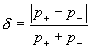(Eq. 7)

3.5
In practice, we first randomly draw opinions of our population from a uniform distribution. Then we initialise the Np+ most positive opinions and Np- most negative opinions with the ue uncertainty, and the others with the uncertainty u.

#### The attractors: bipolarisation or single polarisation

3.6
This very simplified model of extremism, exhibits different dynamical regimes. One would a priori expect a couple of possible regimes: either attraction by the extremes, or central clustering. In fact, we observed parameter zones in which the extremists have a small influence on the rest of the population, and other zones where on the contrary, the majority of the population becomes extremist, either in both extremes or, in one single extreme.

3.7
The following set of figures, obtained from numerical situation, exhibits the three different dynamical regimes. The x-axis codes for time (number of iterations), the y-axis for opinions, and the colours for uncertainty. Each trajectory allows following the evolution in opinion and uncertainty of one individual agent. Common parameters are, µ = 0.5, delta = 0, ue = 0.1, N = 200. The initial uncertainty parameter u of the general population is increased from figure 5 to 7.

3.8
Figure 5, obtained for u=0.4, shows an example of central convergence. In this case, only a marginal part of the initially non-extremists became extremist (4%).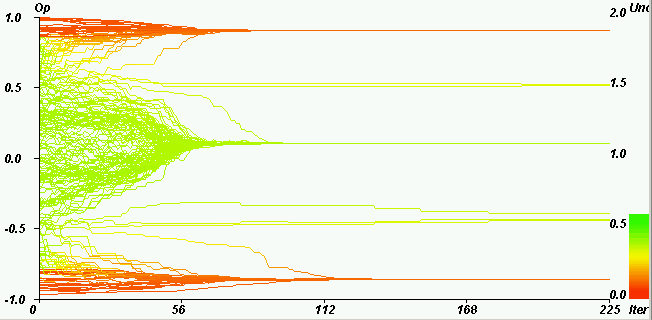Figure 5. Example of central convergence. Horizontal axis: iterations. Vertical axis: opinions. Coloured axis: uncertainties. pe = 0.2, u = 0.4, µ = 0.5, delta = 0, ue = 0.1, N = 200. The majority (96%) of the moderate agents (initially green, between the two extremes) are not attracted by the extremes. The convergence indicator value (defined in section 4) is 0.03.

3.9
Figure 6, obtained for u = 1.2 shows an example of bipolarisation. In this case, the moderate agents are attracted by one of the extremes according to their initial position.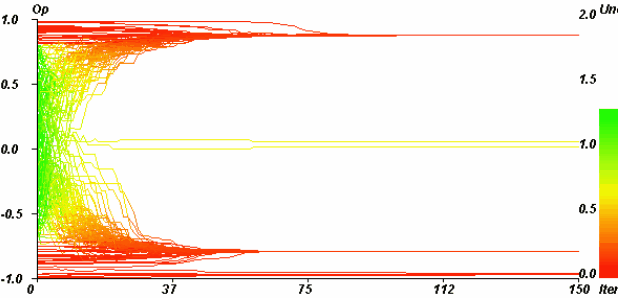Figure 6. Example of both extremes convergence. Horizontal axis: iterations. Vertical axis: opinions. Coloured axis: uncertainties. pe = 0.25, u = 1.2, µ = 0.5, delta = 0, ue = 0.1, N = 200. The initially moderate agents (initially green, between the two extremes) split and become extremists (43% on the positive side, 56% on the negative side). The convergence indicator value (defined in section 4) is 0.49.

3.10
Figure 7 obtained for u = 1.4 shows an example of single polarisation. In this case, the majority of the population is attracted by one of the extremes. This behaviour can take place even when the number of initial extremists is the same at both extremes, which was a priori unexpected.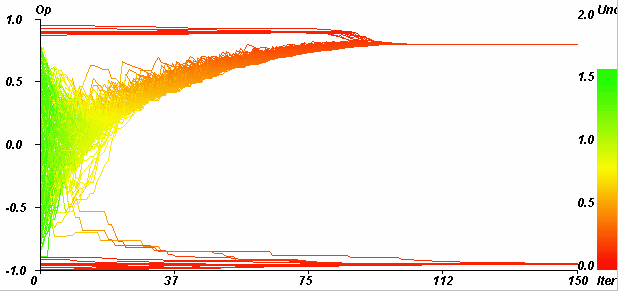Figure 7. Example of single extreme convergence. Horizontal axis: iterations. Vertical axis: opinions. Coloured axis: uncertainties. pe = 0.1, u = 1.4, µ = 0.5, delta = 0, ue = 0.1, N = 200. The majority (98.33%) of initially moderate agents (initially green, between the two extremes) is attracted by the negative extreme. The convergence indicator value is 0.97.

3.11
For another sample drawn from the same initial distribution, all other parameters being equals, one can observe a central convergence (see figure 8). This dependence of which attractor is reached upon initial conditions is a signature for instability.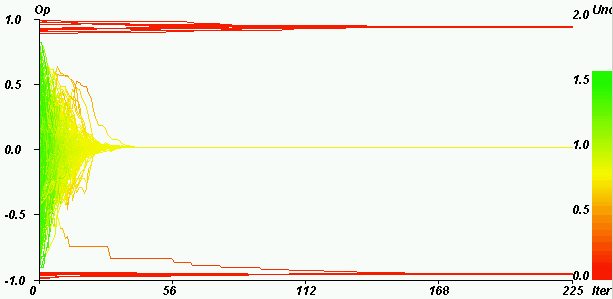Figure 8. Central convergence for the same parameters as in figure 7. Horizontal axis: iterations. Vertical axis: opinions. Coloured axis: uncertainties. pe = 0.1, u = 1.4, µ = 0.5, delta = 0, ue = 0.1, N = 200. The majority stays at the centre (Only one agent joins the negative extreme). The convergence indicator value is 3.0 e-5.

3.12
This fact (instability of either attractor) is confirmed by the master equation analysis. (Faure et al. 2002) derived the master equation corresponding to the RA model and also studied its dynamical behaviour in the same parameter regions. For a perfect initial uniform distribution of opinions, the master equation (which is deterministic) displays a symmetric attractor (either central or on both extremes) in the region where single extreme convergence is obtained with the multi-agent RA model. But any slight asymmetry in the initial distribution changes the central convergence into a single sided extreme attractor (which side is determined by the asymmetry).

3.13
The colour coding of the uncertainties shows that in all three cases clustering not only occurs among the opinions but also among uncertainties: e.g. when extremism prevails, it prevails in both opinions and uncertainties.

3.14
We also ran systematic simulations of the BC model, to compare with the RA model, and in particular to check the genericity of asymmetric extreme attractors. The main result is that single extreme convergence is obtained with the BC model, but the convergence to both extremes is obtained only for a very specific value of the general uncertainty U (see further in section 6 for more details).

3.15
The results of a more complete exploration of the RA model in the parameter space are described in the next section.

###General results of the parameter space exploration

#### Convergence type indicator

4.1
We expressed the results of the exploration with an indicator of the convergence type, denoted y. To compute indicator y, we consider the population of opinions after convergence, and:
• we compute the proportions p'+ and of the initially moderate agents which became extremists in the positive extreme or negative extreme.
• The indicator is defined by: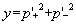(Eq. 8)

4.2
The value of this indicator indicates the type of convergence (summarised in table 1): If none of the moderate agents becomes extremist, then p'+ and p'- are null and y = 0. If half of the initially moderate converge to the positive extreme and half to the negative one, we have p'+ = 0.5 and p'- = 0.5, and therefore y = 0.5. If all the moderate agents go to only one extreme (say the positive one), we have p'+ =1 andp'- = 0, and therefore y = 1.
Table 1: interpretation of the values of y. The intermediate values correspond to intermediate convergence.
 The intermediate values of the indicator correspond to intermediate situations. Value of y 0 0.5 1 Type of convergence Central Both extremes Single extreme

#### Typical patterns of y

4.3
We found that the exploration of the model can be conveniently presented as variations of y with U and pe. This representation leads to one typical pattern of average y for δ = 0, and another one for δ > 0. There is therefore a significant change between the cases where the proportion of positive and negative extremists is exactly the same, and when it is slightly different. When the other parameters ue and µ are modified, the global shape of the patterns remains similar: only the position of the boundaries between the convergence zones varies.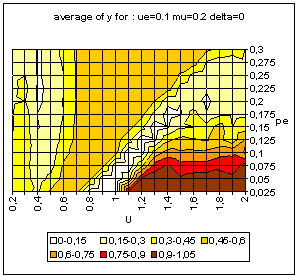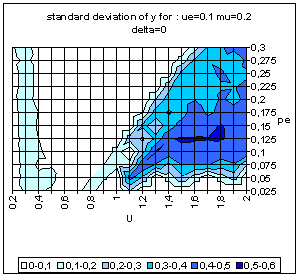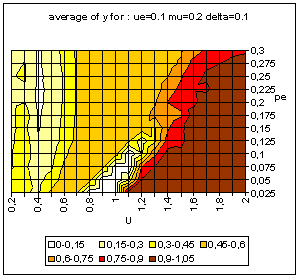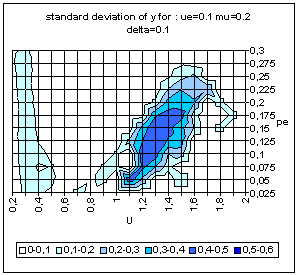Figure 9. Typical pattern of average and standard deviation of indicator y (50 simulations at each point of the graph) as a function of the uncertainty of the moderate agents (U) and the global proportion of extremists (pe) for delta = 0 (top) and delta = 0.1 (bottom). The other parameters are fixed: uncertainty of the extremists ue = 0.1, intensity of interactions µ = 0.2, initial relative difference between the extremists, delta = 0.1. On the graph of average y, the yellow or white zones on the left part correspond to central convergence, the orange, typically in the upper middle part to both extremes, and brown at the bottom right to single extreme.

4.4
The typical patterns obtained for δ = 0 and δ > 0 are shown on figure 9. In this figure, each point of the grid corresponds to 50 simulations with 1000 agents. One can identify 4 regions with different average y values corresponding to the 3 dynamical regimes: two white-yellow zones (one on the left and the other one starting in diagonal from the lower middle part) corresponding to central convergence, one orange zone (drawing a triangle in the middle zone) for double extreme convergence and one brown zone (at the bottom right): single extreme convergence). The dynamical regimes diagrams of figure 9 display large regions of intermediate y values: "pure" dynamical regimes, corresponding to y = 0 or 0.5 or 1, are separated by "crossover" regions where intermediate average y values and high standard deviation could be due to:
• Either a bimodal distribution of "pure" attractors depending on random sampling of initial conditions and pairing;
• Or to a unimodal distribution of more complex attractors with varying number of agents in different clusters.

4.5
The histogram of y values taken along the horizontal line pe = 0.125 (see figure 10) removes the ambiguity about the nature of the attractors in the crossover region. We see that the low U crossover region corresponds to a unimodal distribution y between 0 (central convergence) and 0.5 (both extremes convergence), while the large U crossover corresponds to a bimodal distribution of either central or single extreme convergence.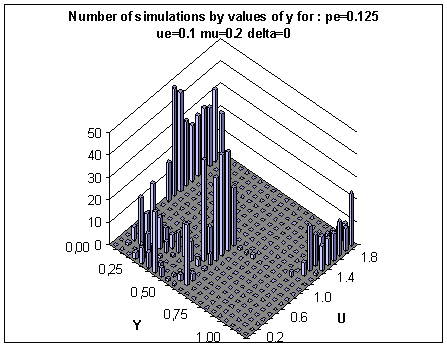Figure 10. Histograms of the values of the y indicator taken along the line pe = 0.125 of figure 9 with δ = 0. In the large u crossover region (u > 1) the same parameters lead to either central or single extreme clustering (y close to 0 or close to 1). In the medium u (0.5 < U < 1) we only get both extremes clustering (y close to 0.5). In the small u crossover region (u below 0.5), the histogram shows different convergences which are intermediary between central and double extremes (with varying proportions of agents having joined the extremes).

4.6
We are now considering how these typical patterns are modified with the variation of other parameters.

#### Influence of the parameter variations on the typical patterns

4.7
We performed a systematic exploration of the parameter space, in order to identify the influence of the different parameters on the type of convergence. All the simulations were repeated 10 times for each set of parameters, which makes a total of 84 000 simulations. All the simulations were performed with a population of 1000 agents.
Table 2: tested values of the parameter space.
DescriptionSymbolTested values
Global proportion of extremistspe0.025, 0.5, 0.1, 0.15, 0.2, 0.25, 0.3
Initial uncertainty of the moderate agentsU0.2, 0.4, 0.6, 0.8, 1, 1.2, 1.4, 1.6,1.8, 2
Initial uncertainty of the extremistsue0.05, 0.1, 0.15, 0.2
Relative difference between the proportion of positive and negative extremists:(Eq. 9)

δ0, 0.1, 0.2, 0.3, 0.4, 0.5
Intensity of interactionsµ0.1, 0.2, 0.3, 0.4, 0.5

4.8
The exploration led to the following results:
• When the intensity of interactions (µ) increases, the both extremes convergence zone increases and the single extreme convergence zone decreases. Moreover, in the case of a same number of extremists on each extreme (δ= 0), the central convergence zone between the both extreme and single extreme convergence zones decreases (see figure 11).
• When the initial bias between the extremists (δ) increases the single extreme convergence zone increases, and the both extremes convergence zone decreases (see figure 12).
• We did not find any significant influence of the uncertainty of the extremists (ue) on the patterns (see figure 13)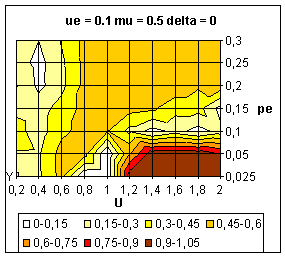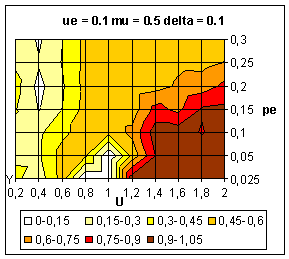Figure 11. Average value of y over 10 simulations. Influence of intensity parameter (µ) on the dynamics. These figures must be compared with figure 9.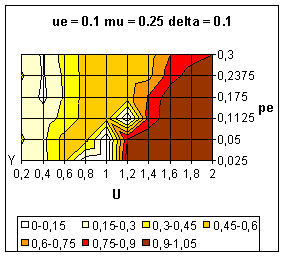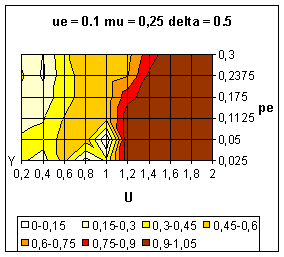Figure 12. Average value of y over 10 simulations. Influence of the bias between the extremes (delta) on the dynamics.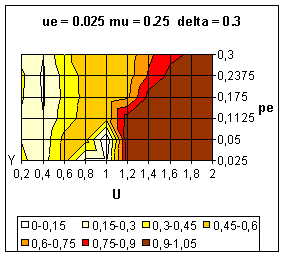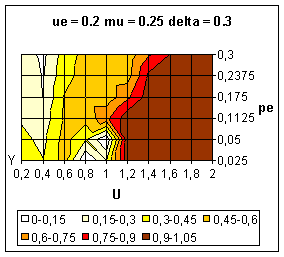Figure 13. Average value of y over 10 simulations. Influence of the extremists' uncertainty (ue) on the dynamics.

###Analysis of the convergence process

5.1
In this section, we propose a deeper analysis of the convergence process on representative examples.

#### Method of analysis

5.2
Our analysis is based on the computation of the mean influence of the population on the opinion of each agent, and the contribution of the extremists to this global mean influence.

5.3
More precisely, for each agent j and for each time step, we compute Ej, which is the mean influence due to the extremists divided by the global mean influence: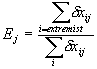(Eq. 10)

5.4
Where is the "virtual" modification of agent j's opinion under the influence of agent i. In fact, at any time step, only one interaction with a randomly chosen agent can actually take place.

5.5
In the following, we represent the mean contribution of the extremists to the global mean influence as a colour, and we artificially bounded the negative values by -1. When δxij values are close to 1, the global mean influence is almost exclusively due to the extremists. When they are close to -1, the extremists have an influence, which is opposite to the global mean influence.

#### Central convergence for initial small uncertainty of the moderate (U)

5.6
Figure 14 shows an example of opinion evolution in the small range of U (U = 0.4), which leads to a central convergence.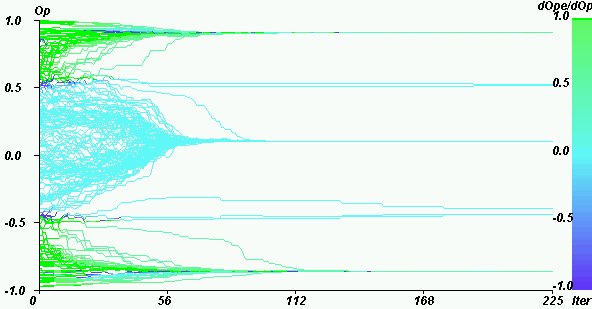Figure 14. Weight of the extremists in the mean influence on the opinion for U = 0.4, pe = 0.2, delta = 0, N = 200. Green points close to the both extremes indicate that the influence is mainly the effect of the extremists. Turquoise points in the middle indicate that the the influence of the extremists is negligible. Blue points, just in between, indicate that the influence of the extremists is contrary to the global influence.

5.7
The figure shows roughly two types of trajectories: the green ones, close to the both extremes, are mainly due to the influence of the extremists, whereas the extremists not at all influence the turquoise trajectories in the middle. At the limit of the extremists influence, there are some dark blue points that indicate that the global mean and the extremists influences are opposite, and can neutralise each other. This explains why a few agents continue horizontally from these zones.

5.8
This situation corresponds to the intuitive case where the extremists attract the parts of the population, which are close to their opinions. In this case, their influence is limited to these initially close parts of the population.

#### Both extremes convergence

5.9
The both extreme convergence can take place with different values of the uncertainty of the initially moderate agents. Figure 15 shows an example of both extremes convergence with a relatively small initial uncertainty of the moderate agents (U = 0.8), and figure 16, a case where this initial uncertainty is higher (U = 1.6).

5.10
One would expect the both extremes convergence to correspond to an extension of the extremist attraction observed on figure 14. The analysis of figure 15 shows that it is not exactly the case. The agents that are mostly attracted by the extremes (green trajectories) have initially medium opinions, and on the contrary, the agents which are the closest to the extremes, tend to be more attracted by the centre (dark blue trajectories).

5.11
The reason is that for a value of the initial moderate uncertainty of 0.8, the agents which are close to the extremes, have a large part of their segment which is out of the width of the initial distribution. There is therefore a higher influence of the central population, which is not compensated by the presence of the extremists. On the contrary, agents with an opinion closer to the centre have the extremists located at one extremity of their segment of uncertainty, and only moderate agents in the rest of the segment. Furthermore, before the final decrease, the attraction gets stronger when the distance between agents increases (see figure 2). The comparatively higher influence of the extremists has a kind of leverage effect, which is determinant.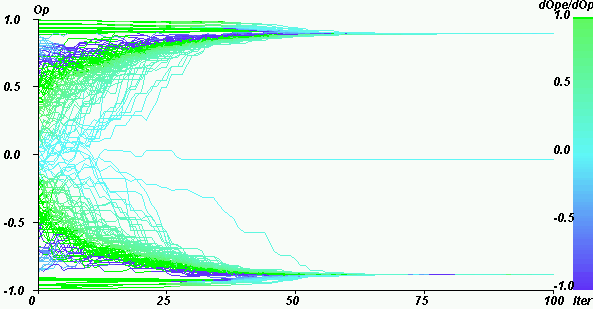Figure 15. Mean influence in a case of both extreme convergence. The values of the parameters are: pe = 0.15, U = 0.8, µ = 0.5, delta = 0, N = 200. We note that the extremists have no significant influence on the closest to the extremes (dark blue points). On the contrary, the extremists have a preponderant influence in a zone of more initially moderate opinions (green parts).

5.12
Figure 16 shows a both extreme convergence for a larger initial uncertainty (and a larger proportion of extremists). One can observe quite different processes:
• At the beginning, the moderate agents tend to cluster at the centre, and the influence of the extremists is small (turquoise green colour).
• Then, in a second phase, there is a strong competition between the influence of the extremists and the influence of the moderate (dark blue parts of trajectories). The reason is that the uncertainties of the agents globally decreased and reached values for which the agents have only one extreme at the extremity of their opinion segment, which enhances the extremists' influence.
• Finally, the extremists win this competition and split the population in two almost equal parts. If the proportion of extremists had been lower, the clustering towards the centre could have prevailed (see next paragraph).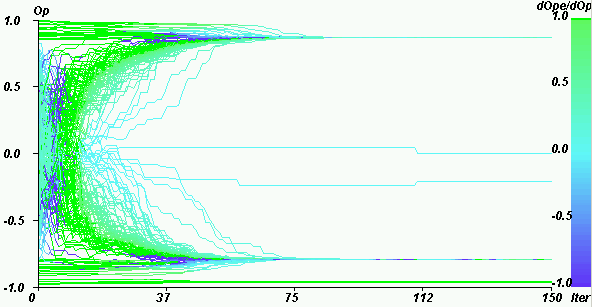Figure 16. Weight of the extremists in the mean influence for U = 1.6, pe = 0.2, µ = 0.5, delta = 0, N = 200. At the very beginning, the extremists have a low influence and the population tends to cluster at the centre. Then, a competition between the extremists' influence and the central clustering tendency occurs (because the uncertainty of the agents decreased).

#### Single extreme convergence

5.13
Figure 17 shows an example of single extreme convergence. In this case, there is a low proportion of extremists, and their role is very small at the very beginning of the process (mostly turquoise trajectories), except at the centre where some green parts appear.

5.14
Then a competition between clustering and dispersion occurs (dark blue parts), which is won by the central clustering process. During this phase a limited number of agents (5) join the extremes.

5.15
One can notice that the process is not perfectly symmetric, because of randomness in the initial distribution and in the sampling of interaction pairs. In particular, at the end of the central clustering phase, there is a bottom part which is turquoise, indicating that the negative extremists are not in contact any more with the central cluster. Therefore, only the positive extremists have an influence on the cluster, and attract it progressively.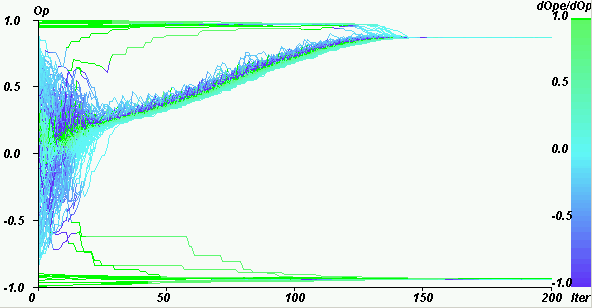Figure 17. Weight of the extremists in the mean influence for U = 1.4, pe = 0.1, µ = 0.5, delta = 0. Case of single extreme convergence.

5.16
During the progressive drift to the positive extreme, we notice that the trajectories can roughly be separated into three parts: green at the bottom, dark blue at the top and middle, and a set of blue turquoise jumps in the upper part (see figure 17). The interpretation is the following:
• The agents, which are at the bottom half of the cluster, tend to go up, because of the influence of the extremists and of the clustering tendency. The weight of the extremists is high because the density is higher at the bottom, and most of the agents are very close to each other. The influence of the majority is therefore low (on average) compared to the influence of the extremists.
• The agents, which are at the top half of the cluster, are submitted to contradictory influences: they tend to go down to the gravity centre of the cluster and the extremists can also attract them up. This is why they are dark blue.
• When the agents are attracted by the extremists and have deviated from the majority, the influence of this majority becomes higher (because they are further), and they tend to go back to the centre of the cluster. However, doing so, they also attract up some agents. This leads to the progressive drift to the extreme (see figure 18).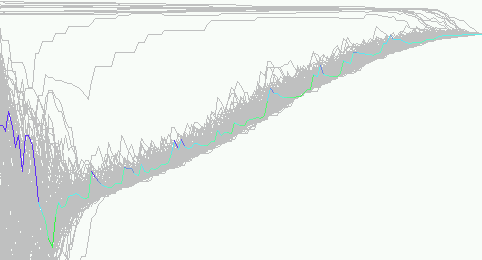Figure 18. Zoom on an individual trajectory in the run of figure 17, all the trajectories are in grey, except one, for which the colour indicate the weight of the extremists in the mean influence. After the central clustering process, the agents fluctuate during the drift to the extreme. From times to times they go suddenly up under the influence of an extremist. Then they go down under the attraction of the close majority. This leads to the progressive drift of the clustered majority to the extreme.

#### Central convergence at the boundary between both extremes and single extreme convergence

5.17
Figure 19 shows an example of central convergence obtained with the same parameters as on figure 17 (Large uncertainty U = 1.4, and a low number of extremists pe = 10%).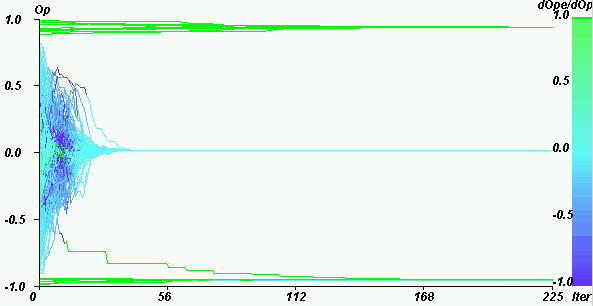Figure 19. Weight of the extremists in the mean influence for U = 1.4, pe = 0.1, µ = 0.5, delta = 0. For the same parameters as on figure 17, we obtain a central convergence.

5.18
We notice a beginning of the process, which is similar to the one of figure 17: initially the extremists have a low influence, and then, when the uncertainty gets smaller, there is a competition between the attraction toward the extremes and toward the centre (dark blue parts). During this phase, one agent actually joins the negative extreme. Finally, the central clustering process wins. In this case, the central cluster is very close to the centre and the contact with the extremists is lost because the uncertainties are too small (the trajectories become turquoise). Therefore, the cluster remains at the centre.

5.19
The fluctuations on the position of the central cluster explain therefore why for the same parameter values, there can be either a single extreme or a central convergence.

###Convergence patterns for BC model variants

6.1
When observing unexpected phenomena, such as the evolution towards single-sided extremism, it is important to check whether the new behaviour is generic, i.e. whether it depends on some specific assumption of our model or whether it can be observed for a large class of models, equivalent at least in terms of this dynamical property. We mentioned earlier that the same dynamics is observed with the master equation formalism of the RA model as soon as any small asymmetry is introduced in the initial conditions; we pursue here our search for genericity by checking three versions of the BC model.

#### The constant uncertainty version

6.2
In its simplest version the BC model varies from the RA model by having a square window interaction function rather than a progressive one: Let x and x' be a pair of opinions, and u' the uncertainty of opinion x'. If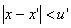the influence of x' on x is given by: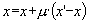(Eq. 11)

6.3
6.3 The uncertainties remain constant throughout the dynamics for all agents. A sample program in C is available as melsimp.c. Figure 20 shows the patterns of results obtained with the constant uncertainty model for populations with extremists and the same set-up that we used with the RA model (figure 9).

6.4
All three types of convergence observed for the RA model also appear with the BC model. However, several major differences with the RA model are noticeable:
• The both extremes convergence takes place only in a very limited parameter zone, with an uncertainty of the population U close to 0.4 or 0.5, corresponding to a convergence to 2 clusters in the case of a population without extremists.
• The single extreme convergence is limited to a zone of parameters around an uncertainty of the population U = 1 (larger for delta = 0.1). In this region, the agents which are attracted by one extreme, loose the contact with the other extreme. Any fluctuation of the density in favour of one or the other extreme leads therefore to the convergence of the whole population to this extreme.
• When U >1.2, we only get central convergence. In this parameter zone, there is a central cluster and some fluctuations of the agents around an average position because of the attraction of the extremists on both sides. However, the moderate agents never go to the extremes.
• The standard deviation of y is high for values which are close to the transition between single extreme convergence and central convergence. There is also a zone of relatively high standard deviation for high percentages of extremists and U around 0.4, because the convergence to both extremes can occasionally take place in this zone.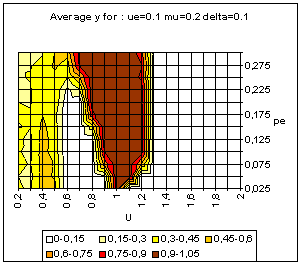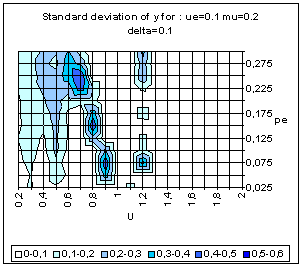Figure 20. Simple BC model. Average and standard deviation of y for 50 simulations on each point of the grid, for delta = 0 (above) and delta = 0.1 (bottom).

#### Bounded confidence with averaging uncertainties

6.5
In this version of the BC model the uncertainties of the two interacting agents get also closer during the interaction according to: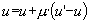(Eq. 12)

6.6
This model is closer to the RA model. Figure 21 displays the dynamical regimes of this model in the same parameter region as figure 9.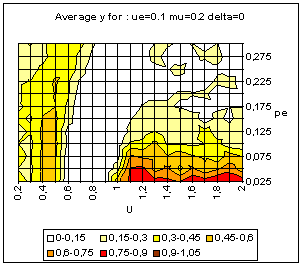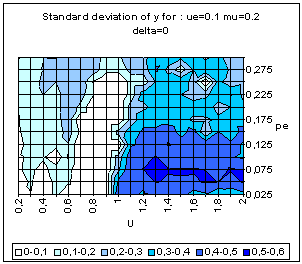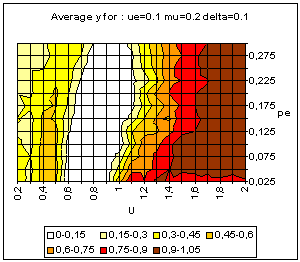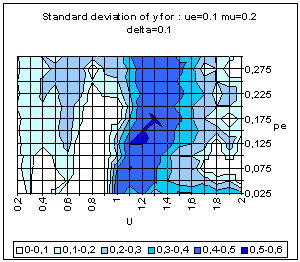Figure 21. BC model with averaging uncertainties. Average and standard deviation of y for 50 simulations on each point of the grid, for delta = 0 (above) and delta = 0.1 (bottom).

6.7
The BC with averaging uncertainties leads also to the three types of convergence. The analysis of figure 21 yields the following noticeable points:
• Like with the simple BC model, the both extremes convergence takes place in a very limited parameter zone (the orange region close to U = 0.4 on the left graphs). Again, it corresponds to the value for which the population converges to 2 clusters in the absence of extremists.
• There is band of central convergence for U close to 0.8. This corresponds to a central clustering which looses the contact with the extremes because of a rapid decreasing of the uncertainty.
• When the initial uncertainty U is higher than 1.1, we tend to get a single extreme convergence or central convergence. If δ > 0, the initial process of central clustering is likely to be closer to one extreme, which leads to a single extreme convergence: the cluster gets closer and closer to the extreme, and looses the contact with the other extreme because the uncertainty decreases. If δ = 0, the central cluster is more likely to be very close to the centre (because of the initial symmetry), and the central convergence is more likely (especially for high percentages of extremists which lead to a faster decrease of the uncertainty and loss of contact with any of the extremes).

#### Bounded confidence with uncertainty based on variance evaluation

6.8
The model of bounded confidence with an evolution of the uncertainty based on variance estimation was proposed in (Weisbuch et al. 2002). The evolution of the opinions and uncertainties is driven by (in a simple version):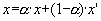(Eq. 13)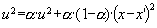(Eq. 14)

6.9
Where α is a positive parameter below 1. This expression gives rise to an approximately exponential decay of uncertainties. As opposed to the BC model with averaging uncertainties, the agents are influenced only by the opinion, and not by the uncertainty of their partner.

6.10
Figure 22 displays the dynamical regimes of this model in the same parameter region as figure 9.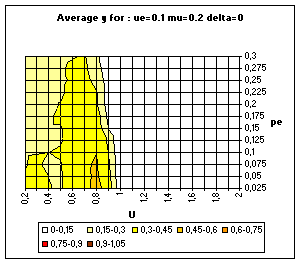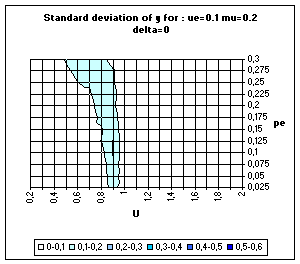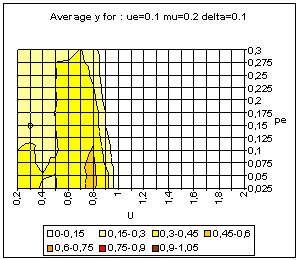Figure 22. BC model with uncertainty based on variance estimation. Average and standard deviation of y for 50 simulations on each point of the grid, for delta = 0 (above) and delta = 0.1 (bottom).

6.11
The striking features of figure 22 are the absence of single extreme convergence, and the very rare presence of double extreme convergence. The reason is the exponential decrease of uncertainty in this model, which rapidly puts the extremists out of uncertainty range of moderate agents.

###Discussion and conclusion

7.1
The behaviour of the relative agreement model can be roughly summarized as follows:
• For small general uncertainty, the influence of the extremists is limited to those agents which were initially close to them, and we get a central convergence.
• For high general uncertainty, the extremes tend to prevail, leading either to a bipolarisation or to the convergence to a single extreme. When the extremists are numerous and of equal number in both extremes, there is an instability between central and single extreme convergence. This instability is due to variations in the position of a central cluster which is formed in a first phase of the process (jointly with a decrease of the uncertainty).

7.2
Some aspects of the RA model seem relevant as a metaphor for social dynamics. The fact that extremism tends to prevail more easily when the population is initially very uncertain corresponds to common sense expectations. More specifically, in the case of central convergence with small initial uncertainties, the attraction by the extremes of those agents initially closer to the extremes was also expected.

7.3
Other aspects of the RA behaviour are more surprising. The fact that the single extreme convergence occurs after a phase of strong consensus at a moderate opinion, with an almost equal interest for both extremes is somehow less intuitive. Moreover, in the case of large uncertainties, it is rather the more central agents which tend to drive the drift to the extremes, whereas the closest to the extremes tend to go to the centre. This was not expected either.

7.4
Comparing the RA to other variants of the BC model shows that the bipolarisation to the extremes is specific to the RA model. The evolution towards single sided extremism is also exhibited both variants of the BC model. The genericity of this dynamical behaviour is a significant result of this analysis.

7.5
Future work is necessary for assessing how these behaviours could correspond to observed social processes, and to assess the limitative character of the many approximations of the models, among which:
• All the agents can communicate with each other (no specific social networks); different structures of social networks can modify the convergence and result in more clusters.
• Agents' opinions are disconnected from any specific information about "the real world out there" and from any strictly personal interest (economic for instance).
• We have not taken into account any power structure or any competition in this power structure, which can have a dramatic impact on the influence of extremism.

###Acknowledgements

We thank David Neau, Edmund Chattoe, Rainer Hegselmann and Nigel Gilbert for helpful discussions and the anonymous referees who made a number of helpful suggestions. We acknowledge the support of the FET-IST department of the European Community Grant IST-2001-33555 COSIN.

###Notes

1This work was originally related to the EU funded IMAGES project (FAIR 3 96 2092), modelling the diffusion of green practises in the European farming population. See (Deffuant 2001) for information.

2Integer and vector opinions dynamics have also been studied in the literature, especially as "cultural dynamics", see e.g. Axelrod R. (1997) "Disseminating cultures" pp. 145-177, in Axelrod R., The complexity of cooperation, Princeton University Press.

3We don't use here uncertainty with the idea that it represents some maximum discrepancy between the agent opinion and some objective reality as it is done in the literature on information cascade. We here use uncertainty since agents with high uncertainty easily change opinion (while those with low uncertainty on the contrary almost never change their opinion). However, this choice is not totally satisfactory because the interpretation of the model includes also the notions of conviction, confidence, tolerance or openness to others influence. Unfortunately, there is no word mixing together all these notions, we chose the one which appeared the most general. Since the model is explicitly defined here, we expect that wording is of less importance than in the traditional sociological literature.

4A difference with the approach of Hegselmann and Krause (2002) and our is that they use a parallel updating of opinions as if at each time step, all agents would survey the opinions of all the others to whom they are connected and average over these opinions. We use random sequential updating based on the idea of pair interactions. Several variants can be imagined for the updating process, random sequential updating seeming a natural one. The only rationales we can imagine for parallel updating is that at the origin (Chatterjee and Seneta 1977; Cohen et al. 1986) the model was built to describe decisions in committees and that the linear (no threshold) model is soluble via matrix techniques.

###References

CHATTERJEE, S. and SENETA, E. 1977. Toward consensus: some convergence theorems on repeated averaging. Journal of Applied Probability, 14, 89-97.

COHEN, J., KAJNAL, J. and NEWMAN, C.M. 1986. Approaching consensus can be delicate when positions harden. Stochastic Processes and their Applications, 22, 315-322.

DEFFUANT, G., NEAU, D., AMBLARD, F. and WEISBUCH, G. 2000. Mixing beliefs among interacting agents. Advances in Complex Systems, 3, 87-98.

DEFFUANT, G. 2001. Final report of project FAIR 3 CT 2092. Improving Agri-environmental Policies: A Simulation Approach to the Cognitive Properties of Farmers and Institutions. <http://wwwlisc.clermont.cemagref.fr/ImagesProject/default.asp>

DITTMER, J.C. 2001. Consensus formation under bounded confidence. Nonlinear Analysis, 47, 4615-4621.

FAURE, T., Deffuant, G., Amblard, F. and Weisbuch, G. 2002. Master equation implementation of the relative agreement model: when does extremism prevail? Communication to the Sociophysics Conference. Bielefeld, Germany.

FRIEDKIN, N.E. and JOHNSEN, E.C. 1990. Social influence and opinions. Journal of Mathematical Sociology, 15, 193-206.

FRIEDKIN, N.E. and JOHNSEN, E.C. 1999. Social influence networks and opinion change. Advances in Group Processes, 16, 1-29.

GALAM, S. 1997. Rational Group Decision Making: a random field Ising model at T=0. Physica A, 238, 66-80.

GALAM, S. and WONCZAK, S. 2000. Dictatorship from Majority Rule Voting. European Physical Journal B, 18, 183-186.

HEGSELMANN, R. and KRAUSE, U. 2002. Opinion Dynamics and Bounded Confidence Models, Analysis and Simulation. Journal of Artificial Societies and Social Simulation, 5, 3. <https://www.jasss.org/5/3/2.html>

KACPERSKY, K. and HOLYST, J. 2000. Phase transition as a persistent feature of groups with leaders in models of opinion formation. Physica A, 287, 631-643.

KRAUSE, U. 2000. A discrete nonlinear and non-autonomous model of consensus formation. In: S.Elaydi, G.Ladas, J.Popenda, J.Rakowski (eds.), Communications in Difference Equations. Amsterdam: Gordon and Breach, 227-236.

LATANE, B. and NOWAK, A. 1997. Self-Organizing Social Systems: Necessary and Sufficient Conditions for the Emergence of Clustering, Consolidation, and Continuing Diversity. In: G.A. Barnett and F.J. Boster (eds.), Progress in Communication Sciences. Ablex Publishing Corporation, 1-24.

WALLEY, P. 1991. Statistical Reasoning with Imprecise Probabilities. Chapman and Hall, London.

WEISBUCH, G., DEFFUANT, G., AMBLARD, F. and NADAL, J.-P. 2002. Meet, discuss and segregate! Complexity, 7, 3, 55-63.